Custom SearchMultiplication Using Powers of 10 From the law of exponents for multiplication we recall that to multiply two or more powers to the same base we add their exponents. Thus, l04 x 102 = 106 We see that multiplying powers of 10 together is an application of the general rule. This is demonstrated in the following examples: 1.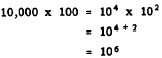2.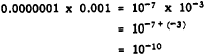3.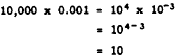4.Therefore,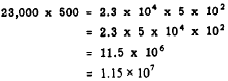5.Therefore,Practice problems. Multiply, using powers of 10. For the purposes of this exercise, treat all numbers as exact numbers: 1. 10,000 x 0.001 x 100 2. 0.000350 x 5,000,000 x 0.0004 3. 3,875 x 0.000032 x 3,000,OOO 4. 7,000 x 0.015 x 1.78 Answers: 1. 1.0 x l03 2. 7.0 x 10-1 3. 3.72 x l05 4. 1.869 x l02 Division Using Powers of 10 The rule of exponents for division states that, for powers of the same base, the exponent of the denominator is subtracted from the exponent of the numerator. Thus,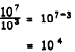It should be remembered that power may be transferred from numerator to denominator or from denominator to numerator by simply changing the sign of the exponent. The following examples illustrate the use of this rule for powers of 10: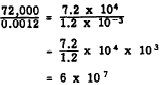2.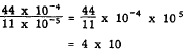Combined Multiplication and Division Using the rules already shown, multiplication and division involving powers of 10 may be combined. The usual method of solving such problems is to multiply and divide alternately until the problem is completed. For example,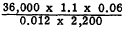Rewriting this problem in scientific notation, we have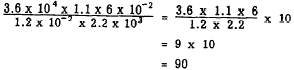Notice that the elimination of O’s, wherever possible, simplifies the computation and makes it an easy matter to place the decimal point. SIGNIFICANT DIGITS.-One of the most important advantages of scientific notation is the fact that it simplifies the task of determining the number of significant digits in a number. For example, the fact that the number 0.00045 has two significant digits is sometimes obscured by the presence of the 0’s. The confusion can be avoided by writing the number in scientific notation, as follows: 0.00045 = 4.5 x 10-4 Practice problems. Express the numbers in the following problems in scientific notation and round off before performing the calculation. In each problem, round off calculation numbers to one more digit than the number of significant digits in the least accurate number; round the answer to the number of significant digits in the least accurate number: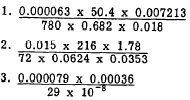Answers: 1. 2.4 x l0-6 2. 3.6 x 10 3. 9.8 x 10-2